This book is in Open Review. I want your feedback to make the book better for you and other readers. To add your annotation, select some text and then click the on the pop-up menu. To see the annotations of others, click the button in the upper right hand corner of the page

## 14.4 Model specification: Outliers

As we discussed in Section ??, one of the important assumptions in forecasting and analytics is the correct specification of the model, which also includes “no outliers in the model” element. Outliers might appear for many different reasons:

1. We missed some important information (e.g. promotion) and did not include a respective variable in the model;
2. There was an error in recordings of the data, i.e. a value of 2000 was recorded as 200;
3. We did not miss anything predictable, we just deal with a distribution with fat tails.

In any of these cases, outliers will impact estimates of parameters of our models. In case of ETS, this will lead to higher than needed smoothing parameters, which leads to wider prediction intervals and potentially biased forecasts. In case of ARIMA, the mechanism is more complicated, but also leads to widened intervals and biased forecasts. So, it is important to identify outliers and deal with them.

### 14.4.1 Outliers detection

One of the simplest ways for identifying outliers is based on distributional assumptions. For example, if we assume that our data follows normal distribution, then we would expect 95% of observations lie inside the bounds with approximately $$\pm 1.96\sigma$$ and 99.8% of them to lie inside the $$\pm3.09 \sigma$$. Sometimes these values are substituted by heuristic “values lying inside 2 / 3 sigmas,” which is not precise and works only for Normal distribution. Still, based on this, we could flag the values outside these bounds and investigate them in order to see if any of them are indeed outliers.

Given that ADAM framework supports different distributions, the heuristics mentioned above is not appropriate. We need to get proper quantiles for each of the assumed distributions. Luckily, this is not difficult to do, because the quantile functions for all the distributions supported by ADAM either have analytical forms or can be obtained numerically.

Here is an example in R with the same multiplicative ETSX model and the standardised residuals vs fitted values with the 95% bounds:

plot(adamModelSeat05, 2, level=0.95)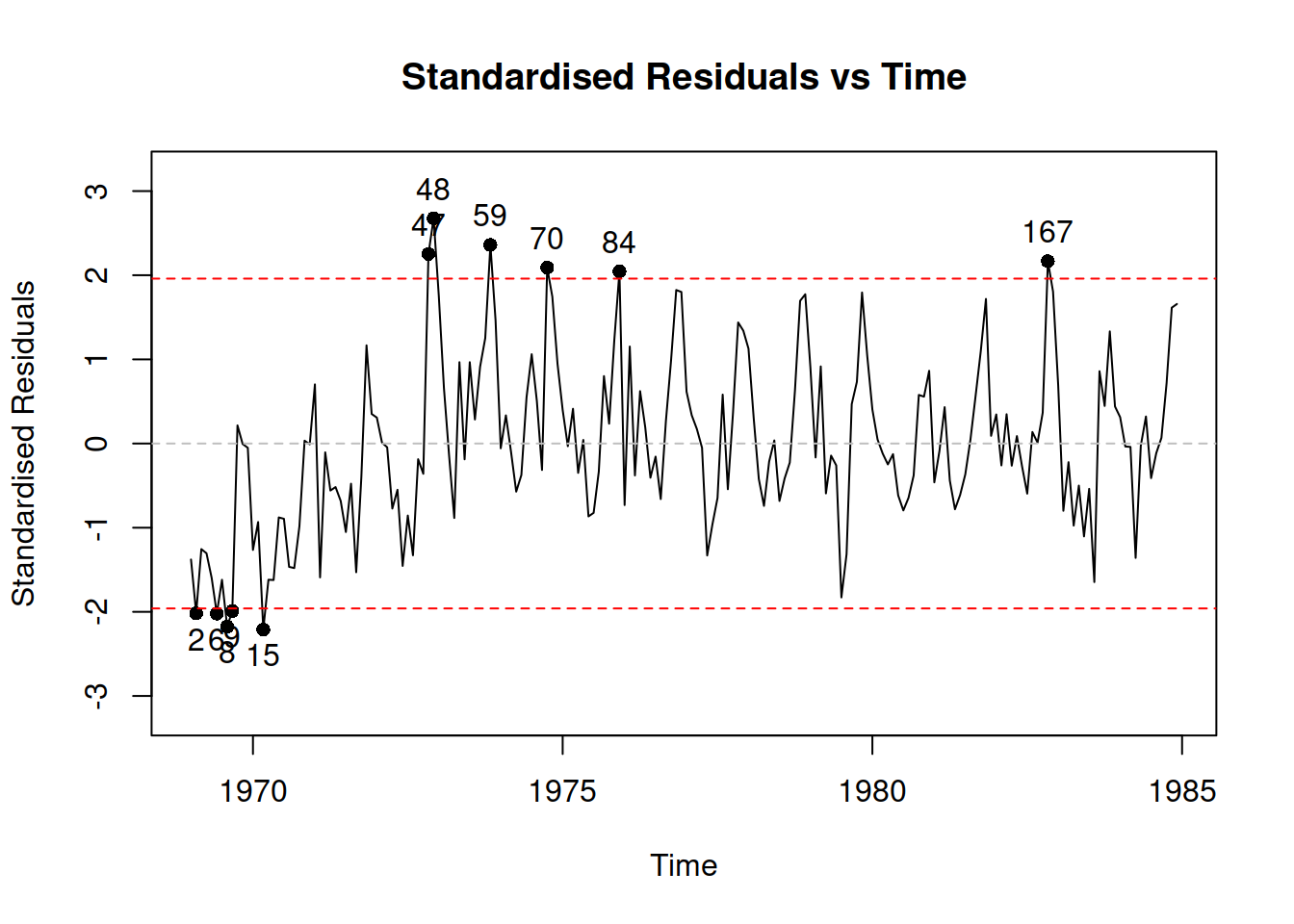Note that in case of $$\mathcal{IG}$$, $$\Gamma$$ and $$\mathrm{log}\mathcal{N}$$, the function will plot $$\log u_t$$ in order to make the plot more readable. The plot demonstrates that there are outliers, some of which are further away from the bounds. Although the amount of outliers is not big, it would make sense investigating why they happened. Well, we know why - we constructed an incorrect model. Given that we deal with time series, plotting residuals vs time is also sometimes helpful:

plot(adamModelSeat05, 8)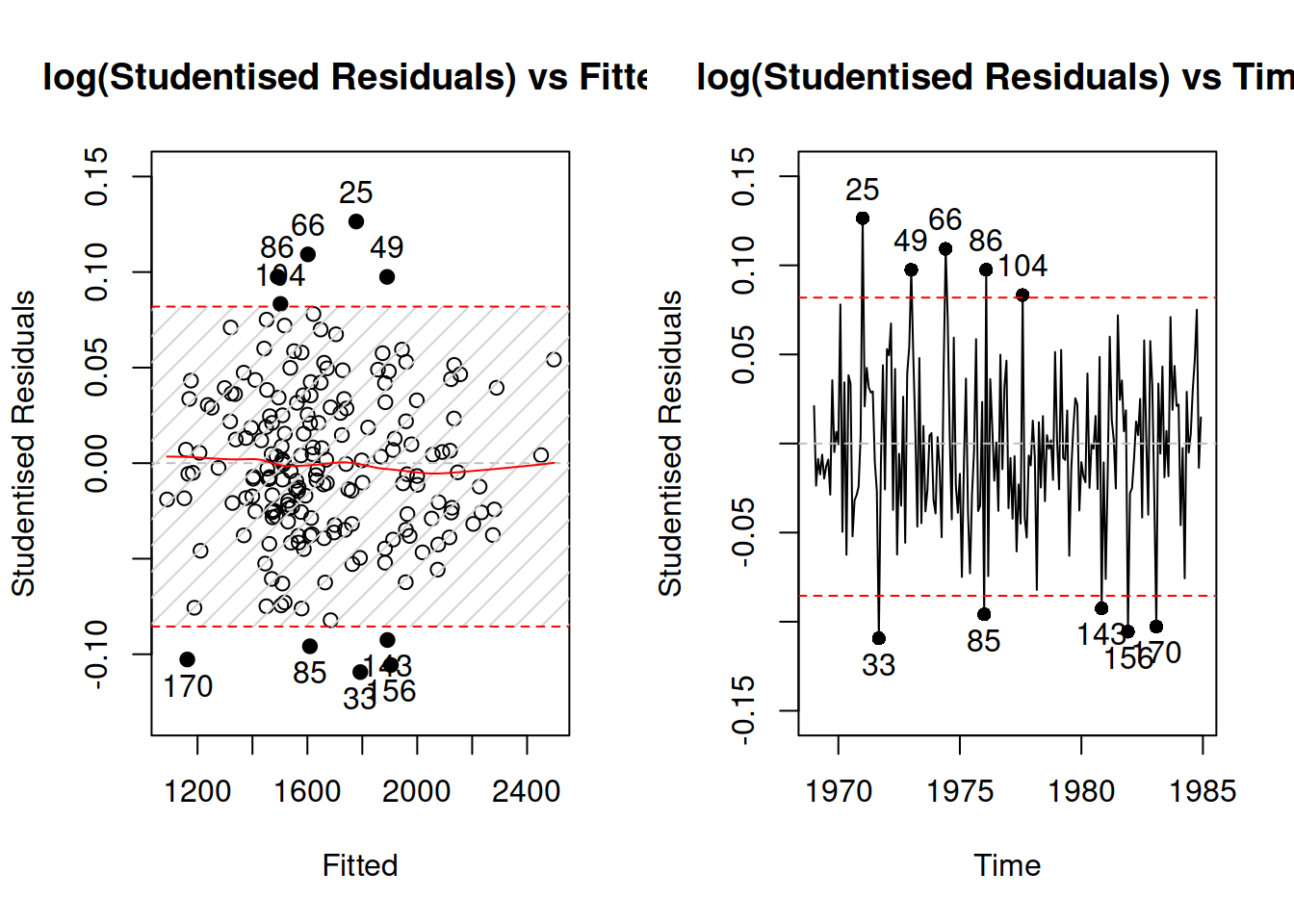We see that there is no specific pattern in the outliers, they happen randomly, so they appear not because of the omitted variables or wrong transformations. We have 5 observations lying outside the bounds, which given that the sample size of 192 observations, means that the 95% interval contains $$\frac{192-9}{192} \times 100 \mathrm{\%} \approx 95.3\mathrm{\%}$$ of observations, which is close to the nominal value.

In some cases, the outliers might impact the scale of distribution and will lead to wrong standardised residuals, distorting the picture. This is where studentised residuals come into play. They are calculated similarly to the standardised ones, but the scale of distribution is recalculated for each observation by considering errors on all but the current observation. So, in a general case, this is an iterative procedure which involves looking through $$t=\{1,\dots,T\}$$ and which should in theory guarantee that the real outliers do not impact the scale of distribution. Here how they can be analysed in R:

par(mfcol=c(1,2))
plot(adamModelSeat05, c(3,9))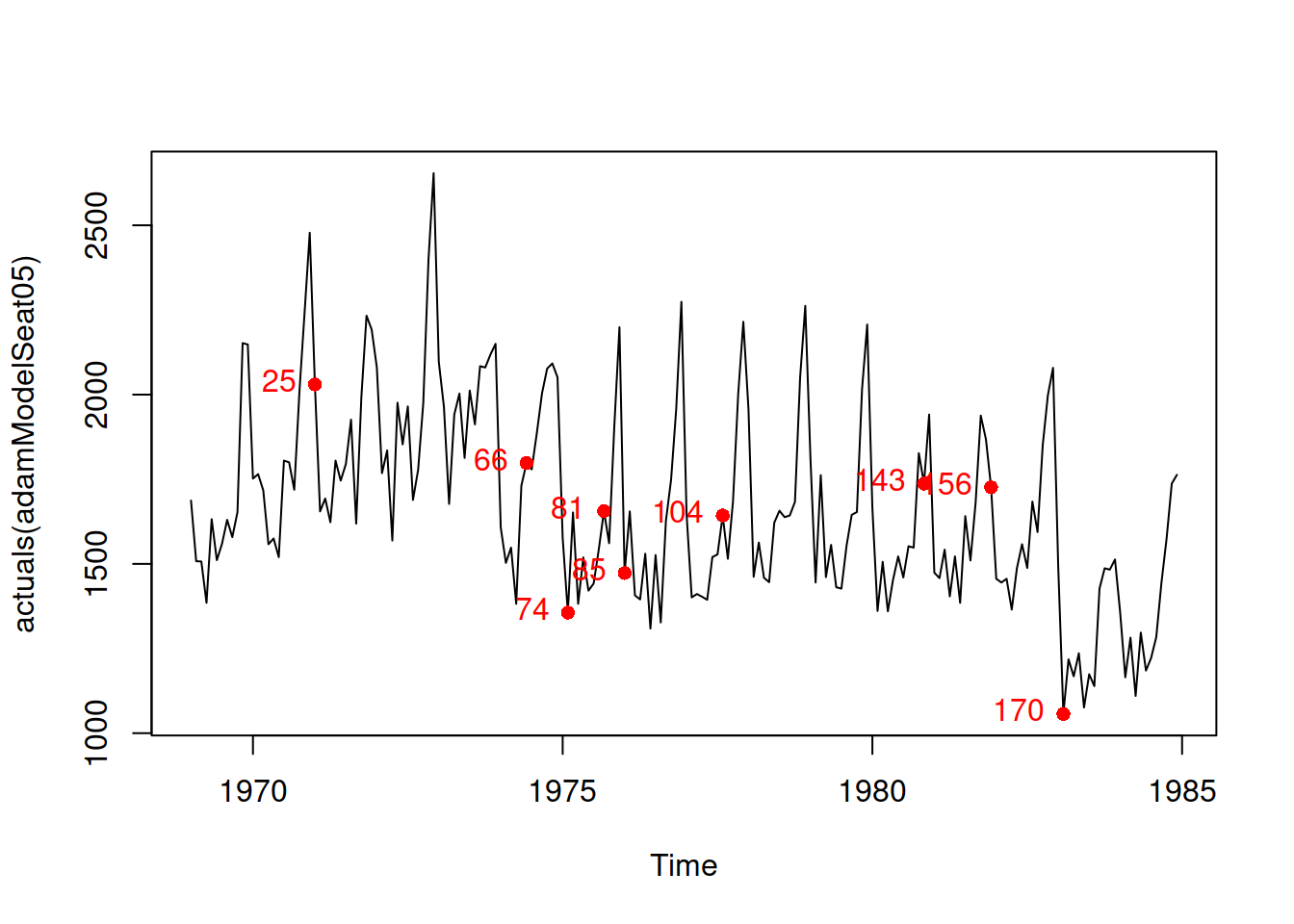In many cases (ours included) the standardised and studentised residuals will look very similar, but in some cases of extreme outliers they might differ and the latter might show outliers better than the former.

Given the situation with outliers in our case, we could investigate when they happen in the original data to better understand whether they need to be taken care of. But instead of manually recording, which of the observations lie beyond the bounds, we can get their ids via the outlierdummy method from the package greybox, which extracts either standardised or studentised residuals and flags those observations that lie outside the constructed interval, automatically creating dummy variables for these observations. Here how it works:

adamModelSeat05Outliers <-
level=0.95, type="rstandard")

The method returns several objects (see documentation for details), including the ids of outliers:

adamModelSeat05Outliers$id ##  25 66 74 81 85 104 143 156 170 These ids can be used to produce additional plots. For example: plot(actuals(adamModelSeat05)) points(time(Seatbelts[,"drivers"])[adamModelSeat05Outliers$id],
Seatbelts[adamModelSeat05Outliers$id,"drivers"], col="red", pch=16) text(time(Seatbelts[,"drivers"])[adamModelSeat05Outliers$id],
Seatbelts[adamModelSeat05Outliers$id,"drivers"], adamModelSeat05Outliers$id, col="red", pos=2)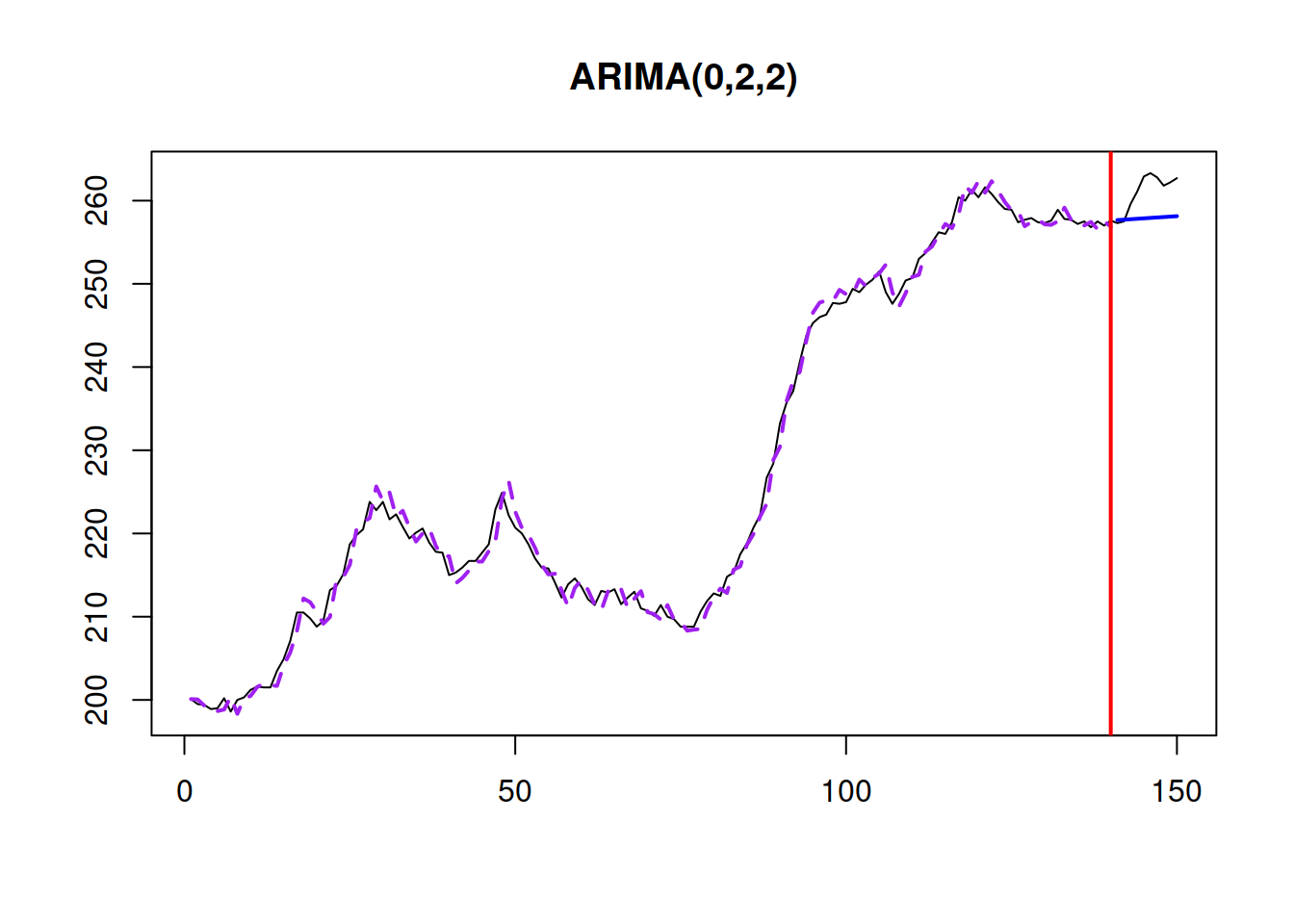Among all these points, there is one special that happens on observation 170. This is when the law for seatbelts was introduced and the model cannot capture the change in injuries and deaths correctly.

Remark. As a side note, in R, there are several methods for extracting residuals:

• resid() or residuals() will extract either $$e_t$$ or $$1+e_t$$, depending on the distributional assumptions of the model;
• rstandard() will extract the standardised residuals $$u_t$$;
• rstudent() will do the same for the studentised ones.

smooth package also introduces rmultistep which extracts multiple steps ahead in sample forecast errors. We do not discuss this method here, but we might come back to it later in this textbook.

### 14.4.2 Dealing with outliers

Based on the output of outlierdummy() method from the previous example, we can construct a model with explanatory variables to interpolate the outliers and neglect their impact on the model:

SeatbeltsWithOutliers <- cbind(as.data.frame(Seatbelts[,-c(1,7)]),
adamModelSeat05Outliers$outliers) SeatbeltsWithOutliers$drivers <- ts(SeatbeltsWithOutliers\$drivers,
start=start(Seatbelts),
frequency=frequency(Seatbelts))
formula=drivers~.)

In order to decide, whether the dummy variables help or not, we can use information criteria, comparing the two models:

setNames(c(AICc(adamModelSeat05), AICc(adamModelSeat06)),
c("ETSX", "ETSXOutliers"))
##         ETSX ETSXOutliers
##     2237.081     2209.273

Comparing the two values above, I would conclude that adding dummies improves the model. But instead of including all of them, we could try the model with the outlier for the suspicious observation 170, which corresponds to the seventh outlier:

adamModelSeat07 <- adam(SeatbeltsWithOutliers, "MNM", lags=12,
formula=drivers~PetrolPrice+kms+
front+rear+law+outlier7)
plot(adamModelSeat07,2)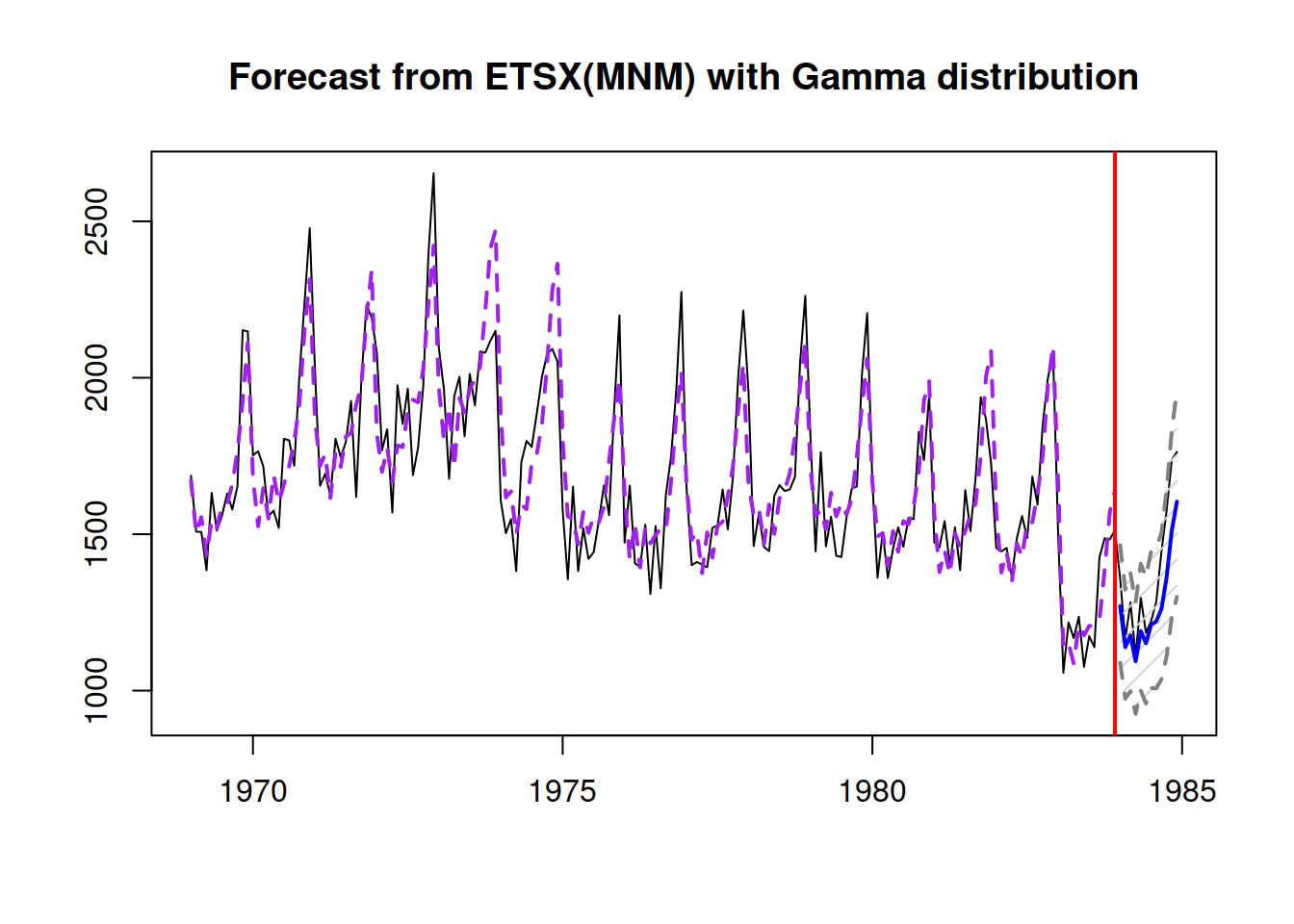AICc(adamModelSeat07)
##  2234.612

This model is slightly worse than both the one with all outliers in terms of AICc, so there are some other dummy variables that improve the fit that might be considered as well, along with the outlier for the observation 170. We could continue the exploration introducing other dummy variables, but in general we should not do that unless we have good reason for that (e.g. we know that something happened that was not captured by the model).

### 14.4.3 An automatic mechanism

A similar automated mechanism is implemented in adam() function, which has outliers parameter, defining what to do with outliers if there are any with the following three options:

1. “ignore” - do nothing;
2. “use” - create the model with explanatory variables as shown in the previous subsection and see if it is better than the simpler model in terms of an information criterion;
3. “select” - create lags and leads of dummies from outlierdummy() and then select the dummies based on the explanatory variables selection mechanism. Lags and leads are needed for cases, when the effect of outlier is carried over to neighbouring observations.

Here how this works for our case:

adamModelSeat08 <- adam(Seatbelts, "MNM", lags=12,
formula=drivers~PetrolPrice+kms+
front+rear+law,
outliers="select", level=0.95)
AICc(adamModelSeat08)
##  3037.296

This automatic procedure will form a matrix that will include original variables together with the outliers, their lags and leads and then select those of them that minimise AICc in a stepwise procedure (discussed in Section 15.3). In our case, the function throws away some of the important variables and sticks with some of outliers. This might also happen because it could not converge to the optimum on each iteration, so increasing maxeval might help. Still, given that this is an automated approach, it is prone to potential mistakes and needs to be treated with care as it might select unnecessary dummy variables and lead to overfitting. I would recommend exploring the outliers manually, when possible and not to rely too much on the automated procedures.

### 14.4.4 Final remarks

explored the question of the impact of outliers on ETS performance in terms of forecasting accuracy. They found that if outliers happen at the end of the time series then it is important to take them into account in a model. If they happen much earlier, then their impact on the final forecast will be negligible. Unfortunately, the authors did not explore the impact of outliers on the prediction intervals, and based on my experience I can tell that the main impact of outliers is on the width of the interval.

### References

• Koehler, A.B., Snyder, R.D., Ord, J.K., Beaumont, A., 2012. A study of outliers in the exponential smoothing approach to forecasting. International Journal of Forecasting. 28, 477–484. https://doi.org/10.1016/j.ijforecast.2011.05.001